# NCERT Solutions for Class 11 Physics chapter-4 Motion In A Plane

## NCERT Solutions for Class 11 Physics

NCERT Solutions for class-11 Physics Chapter-4 Motion In A Plane is prepared by our senior and renowned teachers of pw primary focus while solving these questions of class-11 in NCERT textbook, also do read theory of this Chapter-4 Motion In A Plane while going before solving the NCERT questions. You can download NCERT solution of all chapters from Physics Wallah in PDF.

### Chapter-4 Motion In A Plane

Question1. State, for each of the following physical quantities, if it is a scalar or a vector:
volume, mass, speed, acceleration, density, number of moles, velocity, angular frequency, displacement, angular velocity.

Solution :
Scalar: Volume, mass, speed, density, number of moles, angular frequency
Vector: Acceleration, velocity, displacement, angular velocity

Question2. Pick out the two scalar quantities in the following list:
force, angular momentum, work, current, linear momentum, electric field, average velocity, magnetic moment, relative velocity.

Solution : Work and current are scalar quantities.

Question3. Pick out the only vector quantity in the following list:
Temperature, pressure, impulse, time, power, total path length, energy, gravitational potential, coefficient of friction, charge.

Solution :
Since, Impulse = change in momentum = force × time. As momentum and force are vector quantities, hence impulse is a vector quantity.

Question4. State with reasons, whether the following algebraic operations with scalar and vector physical quantities are meaningful:
b. adding a scalar to a vector of the same dimensions,
c. multiplying any vector by any scalar,
d. multiplying any two scalars,
f. adding acomponent of a vector to the same vector.

Solution :a. Yes, addition of two scalar quantities is meaningful only if they both represent the same physical quantity.
b. No, addition of a vector quantity with a scalar quantity is not meaningful.
c. Yes, scalar can be multiplied with a vector. For example, force is multiplied with time to give impulse.
d. Yes, scalar, irrespective of the physical quantity it represents, can be multiplied with another scalar having the same or different dimensions.
e. Yes, addition of two vector quantities is meaningful only if they both represent the same physical quantity.
f. Yes, component of a vector can be added to the same vector as they both have the same dimensions.

Question5. Read each statement below carefully and state with reasons, if it is true or false:a. The magnitude of a vector is always a scalar,
a. The magnitude of a vector is always a scalar,
b. each component of a vector is always a scalar,
c. the total path length is always equal to the magnitude of the displacement vector of a particle.
d. the average speed of a particle (defined as total path length divided by the time taken to cover the path) is either greater or equal to the magnitude of average velocity of the particle over the same interval of time,
e. Three vectors not lying in a plane can never add up to give a null vector.

Solution :
a. True; because magnitude is a pure number.
b. False, each component of a vector is also a vector.
c. True, only if the particle moves along a straight line in the same direction, if not then it is false.
d. True, because the total path length is either greater than or equal to the magnitude of the displacement vector.
e. True, as they can not be represented by the three sides of a triangle taken in the same order.

Question6. Establish the following vector inequalities geometrically or otherwise:
a. a + b| ≤ a| + b
b. a + b| ≥ |a| − b|
c. a − b| ≤ a| + b
d. a − b| ≥ |a| − b|
When does the equality sign above apply?

Solution :
a. Let two vectors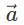and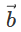be represented by the adjacent sides of a parallelogram OMNP, as shown in the given figure.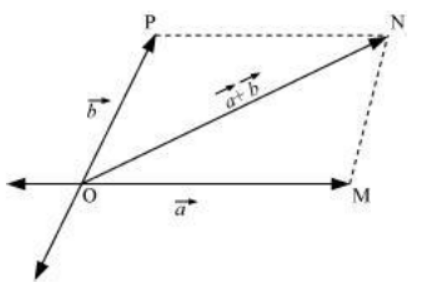Here, we can write: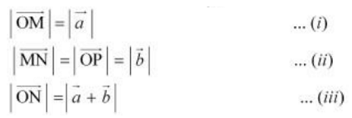In a triangle, each side is smaller than the sum of the other two sides.
Therefore, in ΔOMN, we have:

ON < (OM + MN)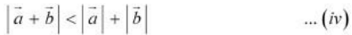If the two vectorsandact along a straight line in the same direction, then we can write: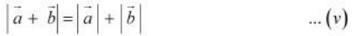Combining equations (iv) and (v), we get: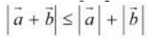b. Let two vectorsandbe represented by the adjacent sides of a parallelogram OMNP, as shown in the given figure.Here, we have:In a triangle, each side is smaller than the sum of the other two sides.

Therefore, in ΔOMN, we have: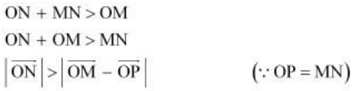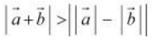… (iv)

If the two vectorsandact along a straight line in the opposite direction, then we can write: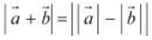… (v)

Combining equations (iv) and (v), we get: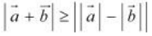c. Let two vectorsandbe represented by the adjacent sides of a parallelogram PORS, as shown in the given figure.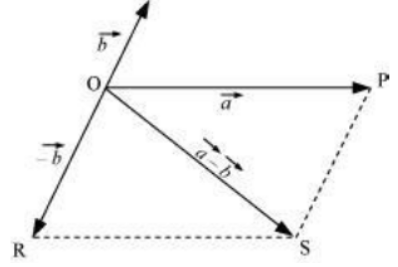Here we have: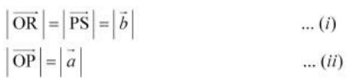In a triangle, each side is smaller than the sum of the other two sides. Therefore, in ΔOPS, we have:
PR < PS + SRIf the two vectors act in a straight line but in opposite directions, then we can write: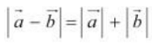… (iv)
Combining equations (iii) and (iv), we get: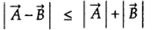d. Let two vectorsandbe represented by the adjacent sides of a parallelogram PORS, as shown in the given figure.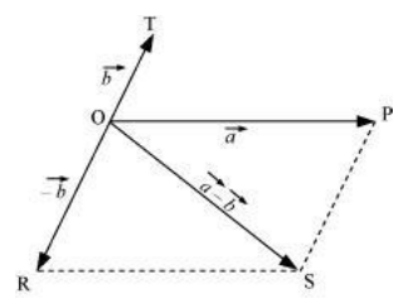The following relations can be written for the given parallelogram.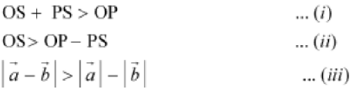The quantity on the LHS is always positive and that on the RHS can be positive or negative. To make both quantities positive, we take modulus on both sides as: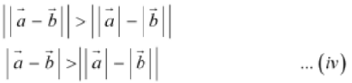If the two vectors act in a straight line but in the same directions, then we can write: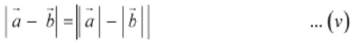Combining equations (iv) and (v), we get: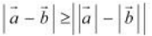Question7. Given vectors a + b + c + d = 0, which of the following statements are correct:
a. Vectors a, b, c, and d must each be a null vector,
b. The magnitude of vectors (a + c) equals the magnitude of vectors(b+ d),
c. The magnitude of a can never be greater than the sum of the magnitudes of b, c, and d,
d. Vectors b + c must lie in the plane of a and d if  a and d are not collinear, and in the line of a and d, if they are collinear?

Solution :
a. In order to make vectors a + b + c + d = 0, it is not necessary to have all the four given vectors to be null vectors. There are many other combinations which can give the sum zero.
b. Correct

a + b + c + d = 0
a + c = – (b + d)
Taking modulus on both the sides, we get:
| a + c | = | –(b + d)| = | b + d
Hence, the magnitude of (a + c) is the same as the magnitude of (b + d).

c. Correct
a + b + c + d = 0
a = - (b + c + d)
Taking modulus both sides, we get:
| a | = | b + c + d |
| a |  ≤  | a | + | b | + | c |  .... (i)
Equation (i) shows that the magnitude of a is equal to or less than the sum of the magnitudes of b, c, and d.
Hence, the magnitude of vector a can never be greater than the sum of the magnitudes of b, c, and d.

d. Correct
For a + b + c + d = 0
a + (b + c) + d = 0
The resultant sum of the three vectors a, (b + c), and d can be zero only if (b + c) lie in a plane containing a and d, assuming that these three vectors are represented by the three sides of a triangle.
If a and d are collinear, then it implies that the vector (b + c) is in the line of a and d. This implication holds only then the vector sum of all the vectors will be zero.

Question8. Three girls skating on a circular ice ground of radius 200 m start from a point Pon the edge of the ground and reach a point Qdiametrically opposite to Pfollowing different paths as shown in Fig. 4.20. What is the magnitude of the displacement vector for each? For which girl is this equal to the actual length of the path skated?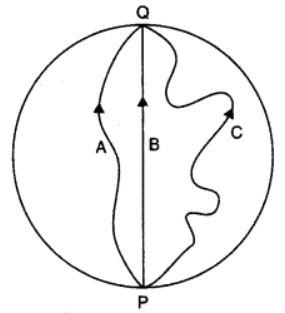Solution :
Displacement is given by the minimum distance between the initial and final positions of a particle. In the given case, all the girls start from point P and reach point Q. The magnitudes of their displacements will be equal to the diameter of the ground.
Radius of the ground = 200 m
Diameter of the ground = 2 × 200 = 400 m
Hence, the magnitude of the displacement for each girl is 400 m. This is equal to the actual length of the path skated by girl B.

Question9. A cyclist starts from the centre Oof a circular park of radius 1 km, reaches the edge Pof the park, then cycles along the circumference, and returns to the centre along QO as shown in Fig. 4.21. If the round trip takes 10 min, what is the

a. net displacement,
b. average velocity, and
c. average speed of the cyclist?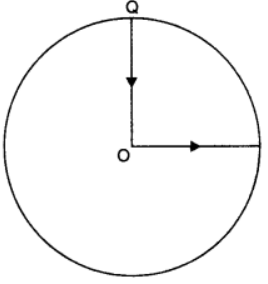Solution :
a. Displacement is given by the minimum distance between the initial and final positions of a body. In the given case, the cyclist comes to the starting point after cycling for 10 minutes. Hence, his net displacement is zero.

b. Average velocity is given by the relation:
Average velocity = Net Displacement / Total time
Since the net displacement of the cyclist is zero, his average velocity will also be zero.

c. Average speed of the cyclist is given by the relation:
Average speed = Total path length / Total time
Total path length = OP + PQ + QO
= 1 + (1 (2π × 1) / 4 )  + 1
= 2 + (π / 2)
= 3.570 km
Time taken = 10 min = 10 / 60 = 1 / 6 h
∴ Average speed = 3.570 / (1/6) = 21.42 km/h

Question10. On an open ground, a motorist follows a track that turns to his left by an angle of 60° after every 500 m. Starting from a given turn, specify the displacement of the motorist at the third, sixth and eighth turn. Compare the magnitude of the displacement with the total path length covered by the motorist in each case.

Solution :

The path followed by the motorist is a regular hexagon with side 500 m, as shown in the given figure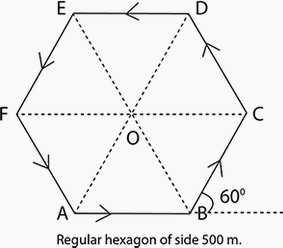The path followed by the motorist is a regular hexagon with side 500 m, as shown in the given figure
Let the motorist start from point A.
The motorist takes the third turn at D.
Total path length =AB+BC+CD=500+500+500=1500m
The motorist takes the sixth turn at point A, which is the starting point.
Magnitude of displacement =0
Total path length =6AB
=6×500=3000m
The motorist takes the eight turn at point C
The magnitude of displacement =AC
From triangle law, the magnitude of displacement is 866.03m at an angle of 30 with AC.
It means AC makes an angle 30 with the initial direction. Total path length =8×500=4000m

Question11. A passenger arriving in a new town wishes to go from the station to a hotel located 10 km away on a straight road from the station. A dishonest cabman takes him along a circuitous path 23 km long and reaches the hotel in 28 min. What is (a) the average speed of the taxi, (b) the magnitude of average velocity? Are the two equal?

Solution :
(a) Total distance travelled = 23 km
Total time taken = 28 min = 28 / 60 h
∴  Average speed of the taxi = Total disttance travelled / Total time taken
= 23 / (28/60) = 49.29 km/h

(b) Distance between the hotel and the station = 10 km = Displacement of the car
∴ Average velocity = 10 / (28/60) = 21.43 km/h
Therefore, the two physical quantities (averge speed and average velocity) are not equal.

Question12. Rain is falling vertically with a speed of 30 m s-1. A woman rides a bicycle with a speed of 10 m s-1 in the north to south direction. What is the direction in which she should hold her umbrella ?

Solution :
The described situation is shown in the given figure.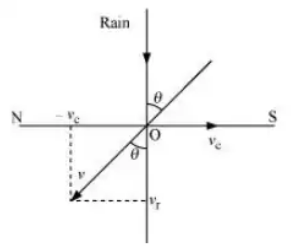Here,

vc = Velocity of the cyclist
vr = Velocity of falling rain
In order to protect herself from the rain, the woman must hold her umbrella in the direction of the relative velocity (v) of the rain with respect to the woman.
v = vr + (-vc)
= 30 + (-10) = 20 m/s
tan θ = vc / vr = 10 / 30
θ = tan-1 (1 / 3)
= tan-1 (0.333) ≈ 180
Hence, the woman must hold the umbrella toward the south, at an angle of nearly 18° with the vertical.

Question13. A man can swim with a speed of 4.0 km/h in still water. How long does he take to cross a river 1.0 km wide if the river flows steadily at 3.0 km/h and he makes his strokes normal to the river current? How far down the river does he go when he reaches the other bank?

Solution :
Speed of the man, vm = 4 km/h
Width of the river = 1 km
Time taken to cross the river = Width of the river / Speed of the river
= 1/4 h = 1 × 60 / 4 = 15 min
Speed of the river, vr = 3 km/h
Distance covered with flow of the river = vr × t
= 3 × 1 / 4 = 3 / 4 km
= 3 × 1000 / 4
= 750 m

Question14. In a harbor, wind is blowing at the speed of 72 km/h and the flag on the mast of a boat anchored in the harbor flutters along the N-E direction. If the boat starts moving at a speed of 51 km/h to the north, what is the direction of the flag on the mast of the boat ?

Solution :
The flag is fluttering in the north-east direction. It shows that the wind is blowing toward the north-east direction. When the ship begins sailing toward the north, the flag will move along the direction of the relative velocity (vwb) of the wind with respect to the boat.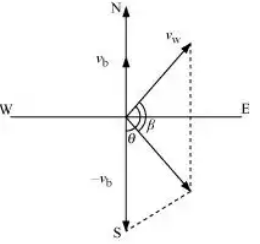The angle between vw and (–vb) = 90° + 45°
tan β = 51 Sin (90 + 45) / (72 + 51 Cos (90 + 45)
Substituting and solving we get,
tan β = 51 / 50.80 = 1.0038
∴ β = tan-1 (1.0038) = 45.110
Angle with respect to the east direction = 45.11° – 45° = 0.11°
Hence, the flag will flutter almost due east.

Question15. The ceiling of a long hall is 25 m high. What is the maximum horizontal distance that a ball thrown with a speed of 40 m s-1 can go without hitting the ceiling of the hall ?

Solution :
Maximum height, h = 25 m
In projectile motion, the maximum height reached by a body projected at an angle θ, is given by the relation:
h = u2 Sin2θ / 2g
25 = (40)2 Sin2θ / 2 × 9.8
sin2 θ = 0.30625
sin θ = 0.5534
∴ θ = sin–1(0.5534) = 33.60°
Horizontal range, R = u2 Sin 2θ / g
= (40)2 × Sin (2 × 33.600) / 9.8
= 1600 × Sin (67.2) / 9.8
= 1600 × 0.922 / 9.8  =  150.53 m

Question16. A cricketer can throw a ball to a maximum horizontal distance of 100 m. How much high above the ground can the cricketer throw the same ball ?

Solution :
Maximum horizontal distance, R = 100 m
The cricketer will only be able to throw the ball to the maximum horizontal distance when the angle of projection is 45°, i.e., θ = 45°.
The horizontal range for a projection velocity v, is given by the relation:
R = u2 Sin 2θ / g
100 = u2 Sin 900 / g
u2 / g = 100   ....(i)
The ball will achieve the maximum height when it is thrown vertically upward. For such motion, the final velocity v is zero at the maximum height H.
Acceleration, a = –g
Using the third equation of motion:
v2 - u2 = -2gH
H = u2 / 2g  =  100 / 2  =  50 m

Question17. A stone tied to the end of a string 80 cm long is whirled in a horizontal circle with a constant speed. If the stone makes 14 revolutions in 25 s, what is the magnitude and direction of acceleration of the stone?

Solution :
Length of the string, l = 80 cm = 0.8 m
Number of revolutions = 14
Time taken = 25 s
Frequency, v = Number of revolutions / Time taken  =  14 / 25 Hz
Angular frequency, ω = 2πν
= 2  × (22/7)  ×  (14/25)  =  88 / 25 rad s-1
Centripetal acceleration, ac = ω2r
= (88 / 25)×  0.8
= 9.91 ms-2
The direction of centripetal acceleration is always directed along the string, toward the centre, at all points.

Question18. An aircraft executes a horizontal loop of radius 1.00 km with a steady speed of 900 km/h. Compare its centripetal acceleration with the acceleration due to gravity.

Solution :
Radius of the loop, r = 1 km = 1000 m
Speed of the aircraft, v = 900 km/h = 900  ×  5 / 18  =  250 m/s
Centripetal acceleration, ac = v2 / r
= (250)2 / 1000 = 62.5 ms-2
Acceleration due to gravity, g = 9.8 m/s2

ac / g  =  62.5 / 9.8  =  6.38
ac =  6.38 g

Question19. Read each statement below carefully and state, with reasons, if it is true or false:
(a) The net acceleration of a particle in circular motion is always along the radius of the circle towards the centre
(b) The velocity vector of a particle at a point is always along the tangent to the path of the particle at that point
(c) The acceleration vector of a particle in uniform circular motion averaged over one cycle is a null vector

Solution :
(a) False, the net acceleration of a particle is towards the centre only in case of a uniform circular motion.
(b) True, because while leaving the circular path, the particle moves tangentially to the circular path.
(c) True, The direction of acceleration vector in a uniform circular motion is directed towards the centre of circular path. It is constantly changing with time. The resultant of all these vectors will be zero vector.

Question20. The position of a particle is given by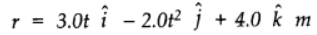where t is in seconds and the coefficients have the proper units for r to be in metres.
a. Find the v and a of the particle?

b. What is the magnitude and direction of velocity of the particle at t = 2.0 s ?

Solution :
a.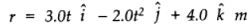The position of the particle is given by: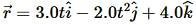Velocity v, of the particle is given as: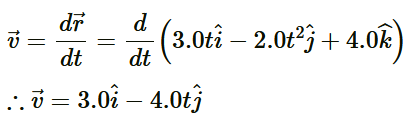Acceleration a, of the particle is given as: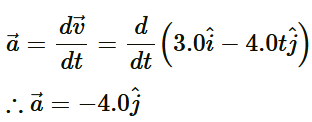b. 8.54 m/s, 69.45° below the x-axis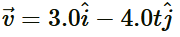The magnitude of velocity is given by: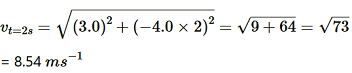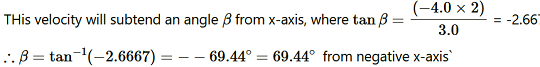The negative sign indicates that the direction of velocity is below the x-axis.

Question21. A particle starts from the origin at t = 0 s with a velocity of 10.0 ĵ m/s and moves in the x-y plane with a constant acceleration of (8.0 î+ 2.0 ĵ) m s-2.

a. At what time is the x- coordinate of the particle 16 m? What is the y-coordinate of the particle at that time?
b. What is the speed of the particle at the time ?

Solution :
(a)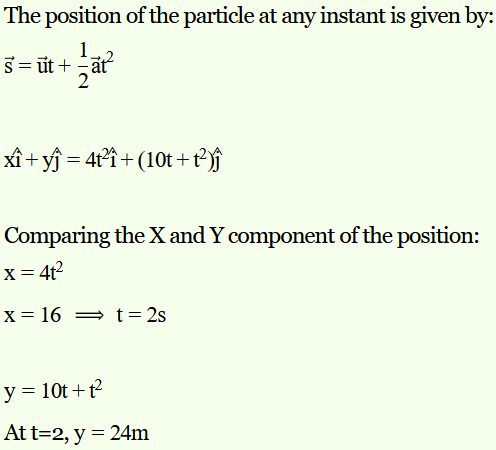(b)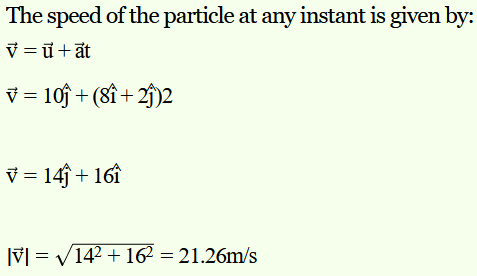Question22. î and ĵ are unit vectors along x and y axis respectively. What is the magnitude and direction of the vectors î + ĵ, and î − ĵ ? What are the components of a vector A= 2î + 3ĵ along the directions of î + ĵ and î − ĵ? [You may use graphical method]

Solution :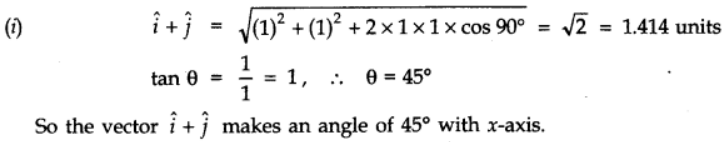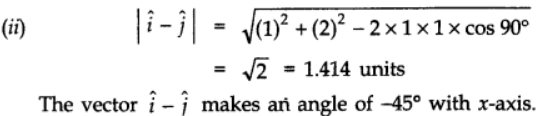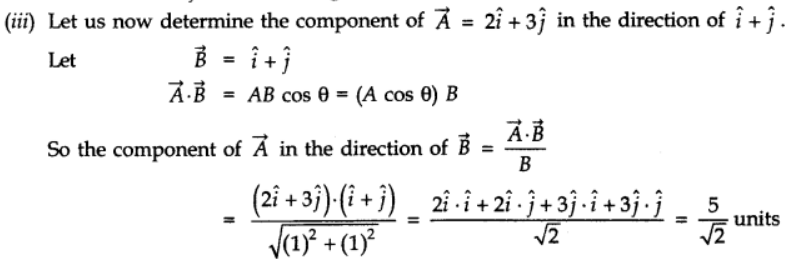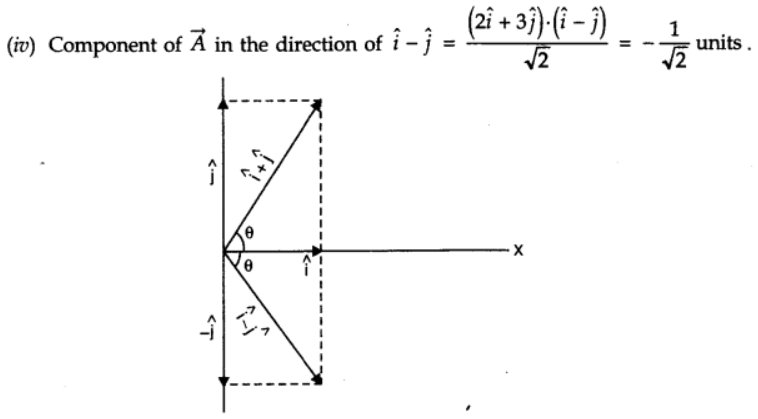Question23. For any arbitrary motion in space, which of the following relations are true :
a. vaverage = (1/2) (v (t1) + v (t2))
b. vaverage = [ r(t2) - r(t1) ] / (t2 – t1)
c. v (t) = v (0) + a t
d. r (t) = r (0) + v (0) t + (1/2) a t2
e. aaverage = [ v (t2) - v (t1 ) ] / ( t2 – t1)
(The ‘average’ stands for average of the quantity over the time interval t1 to t2)

Solution :
a. It is given that the motion of the particle is arbitrary. Therefore, the average velocity of the particle cannot be given by this equation.
b. The arbitrary motion of the particle can be represented by this equation.
c.The motion of the particle is arbitrary. The acceleration of the particle may also be non-uniform. Hence, this equation cannot represent the motion of the particle in space.
d. The motion of the particle is arbitrary; acceleration of the particle may also be non-uniform. Hence, this equation cannot represent the motion of particle in space.
e. The arbitrary motion of the particle can be represented by this equation.

Question24. Read each statement below carefully and state, with reasons and examples, if it is true or false :
A scalar quantity is one that
a. is conserved in a process
b.can never take negative values
c. must be dimensionless
d. does not vary from one point to another in space
e. has the same value for observers with different orientations of axes.

Solution :
a.False
Despite being a scalar quantity, energy is not conserved in inelastic collisions.

b. False
Despite being a scalar quantity, temperature can take negative values.

c. False
Total path length is a scalar quantity. Yet it has the dimension of length.

d.False
A scalar quantity such as gravitational potential can vary from one point to another in space.

e. True
The value of a scalar does not vary for observers with different orientations of axes.

Question25. An aircraft is flying at a height of 3400 m above the ground. If the angle subtended at a ground observation point by the aircraft positions 10.0 s apart is 30°, what is the speed of the aircraft ?

Solution :
The positions of the observer and the aircraft are shown in the given figure.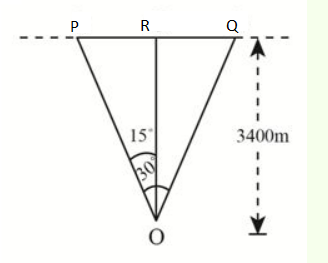Height of the aircraft from ground, OR = 3400 m
Angle subtended between the positions, ∠POQ = 30°
Time = 10 s
In ΔPRO:
tan 150 = PR / OR
PR = OR tan 150
= 3400 X tan 150
ΔPRO is similar to ΔRQO.
∴PR = RQ
PQ = PR + RQ
= 2PR = 2 × 3400 tan 15°
= 6800 × 0.268 = 1822.4 m
∴ Speed of the aircraft = 1822.4 / 10 = 182.24 m/s

Question26. A vector has magnitude and direction. Does it have a location in space ? Can it vary with time ? Will two equal vectors a and b at different locations in space necessarily have identical physical effects ? Give examples in support of your answer.

Solution :
(i) A vector in general has no definite location in space because a vector remains unaffected whenever it is displaced anywhere in space provided its magnitude and direction do not change. However, a position vector has a definite location in space.
(ii) A vector can vary with time as example the velocity vector o an unaffected particle varies with time.
(iii) Two equal vectors at different locations in space do not necessarily have same physical effects. For example, two equal forces acting at two different points on a body which can cause the rotation of a body about an axis will not produce equal turning effect.

Question27. A vector has both magnitude and direction. Does it mean that anything that has magnitude and direction is necessarily a vector ? The rotation of a body can be specified by the direction of the axis of rotation, and the angle of rotation about the axis. Does that make any rotation a vector ?

Solution :
No. A physical quantity having both magnitude and direction need not be considered a vector. For example, despite having magnitude and direction, current is a scalar quantity. The essential requirement for a physical quantity to be considered a vector is that it should follow the law of vector addition.
Generally speaking, the rotation of a body about an axis is not a vector quantity as it does not follow the law of vector addition. However, a rotation by a certain small angle follows the law of vector addition and is therefore considered a vector.

Question28. Can you associate vectors with (a) the length of a wire bent into a loop, (b) a plane area, (c) a sphere ? Explain.

Solution :
(a) No, one cannot associate a vector with the length of a wire bent into a loop.

(b) Yes, one can associate an area vector with a plane area. The direction of this vector is normal, inward or outward to the plane area.
(c) No, one cannot associate a vector with the volume of a sphere. However, an area vector can be associated with the area of a sphere.

Question29. A bullet fired at an angle of 30° with the horizontal hits the ground 3.0 km away. By adjusting its angle of projection, can one hope to hit a target 5.0 km away? Assume the muzzle speed to the fixed, and neglect air resistance.

Solution :
Range, R = 3 km
Angle of projection, θ = 30°
Acceleration due to gravity, g = 9.8 m/s2
Horizontal range for the projection velocity u0, is given by the relation:
R = u02 Sin 2θ / g
3 = u02 Sin 600 / g
u02 / g = 2√3      .......(i)
The maximum range (Rmax) is achieved by the bullet when it is fired at an angle of 45° with the horizontal, that is,
Rmax = u02 / g    ....(ii)
On comparing equations (i) and (ii), we get:
Rmax = 3√3
= 2 X 1.732 = 3.46 km
Hence, the bullet will not hit a target 5 km away.

Question30. A fighter plane flying horizontally at an altitude of 1.5 km with speed 720 km/h passes directly overhead an anti-aircraft gun. At what angle from the vertical should the gun be fired for the shell with muzzle speed 600 m s-1 to hit the plane ? At what minimum altitude should the pilot fly the plane to avoid being hit ? (Take g = 10 m s-2 ).

Solution :
Height of the fighter plane = 1.5 km = 1500 m
Speed of the fighter plane, v = 720 km/h = 200 m/s
Let θ be the angle with the vertical so that the shell hits the plane. The situation is shown in the given figure.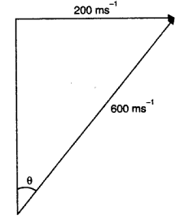Muzzle velocity of the gun, u = 600 m/s
Time taken by the shell to hit the plane = t
Horizontal distance travelled by the shell = uxt
Distance travelled by the plane = vt
The shell hits the plane. Hence, these two distances must be equal.
uxt = vt
u Sin θ = v
Sin θ = v / u
= 200 / 600 = 1/3 = 0.33
θ = Sin-1(0.33) = 19.50
In order to avoid being hit by the shell, the pilot must fly the plane at an altitude (H) higher than the maximum height achieved by the shell.
∴ H = u2Sin2 (90 - θ) / 2g
= (600)2 Cos2 θ / 2g
= 360000 X Cos2 19.5 / 2 X 10
= 16006.482 m
≈ 16 km

Question31. A cyclist is riding with a speed of 27 km/h. As he approaches a circular turn on the road of radius 80 m, he applies brakes and reduces his speed at the constant rate of 0.50 m/s every second. What is the magnitude and direction of the net acceleration of the cyclist on the circular turn ?

Solution :
0.86 m/s2; 54.46° with the direction of velocity
Speed of the cyclist, v = 27 km/h = 7.5 m/s
Radius of the circular turn, r = 80 m
Centripetal acceleration is given as:
ac = v2 / r
= (7.5)2 / 80 = 0.7 ms-2
The situation is shown in the given figure: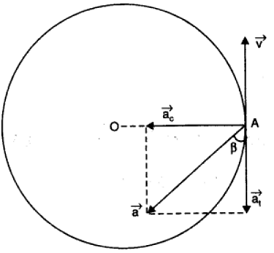Suppose the cyclist begins cycling from point P and moves toward point Q. At point Q, he applies the breaks and decelerates the speed of the bicycle by 0.5 m/s2.

This acceleration is along the tangent at Q and opposite to the direction of motion of the cyclist.
Since the angle between ac and aT is 900, the resultant acceleration a is given by:
a = (ac2 + aT2)1/2
= ( (0.7)2 + (0.5)2 )1/2
= (0.74)1/2 = 0.86 ms-2
tan θ = ac / aT
where θ is the angle of the resultant with the direction of velocity.
tan θ = 0.7 / 0.5 = 1.4
θ = tan-1 (1.4) = 54.560

Question32.a. Show that for a projectile the angle between the velocity and the x-axis as a function of time is given by
θ(t) = tan-1((v0y -  gt) / v0x)
b. Shows that the projection angle θ0 for a projectile launched from the origin is given by
θ0 = tan-1(4hm / R)
where the symbols have their usual meaning.

Solution :
a.  Let v0x and v0y respectively be the initial components of the velocity of the projectile along horizontal (x) and vertical (y) directions.
Let vx and vy respectively be the horizontal and vertical components of velocity at a point P.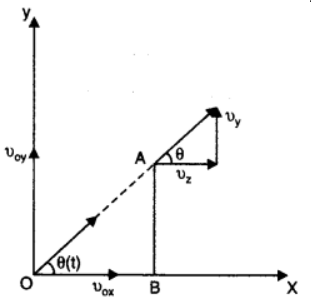Time taken by the projectile to reach point P = t
Applying the first equation of motion along the vertical and horizontal directions, we get:
vy = v0y = gt
And vx = v0x
∴ tan θ = vy / vx = (v0y - gt) / v0x
θ = tan-1 (v0y - gt) / v0x

b. Maximum vertical height, hm = u02 Sin2θ / 2g   ...(i)
Horizontal range, R = u02 Sin22θ / g   ... (ii)
hm / R = Sin2θ / 2Sin2
= Sin θ X Sin θ / 2 X 2Sinθ Cosθ
= Sin θ / 4 Cos θ = tan θ / 4
tan θ = (4hm / R)
θ = tan-1 (4hm / R)